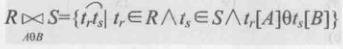## 数据库系统概论--读书笔记--8 关系运算： 选择 投影 连接 除运算

zz/2023/6/4 15:10:50

### 专门的关系运算： 选择 投影 连接 除运算

1.设关系模式为R(A1,A2,⋯&ThinSpace;,An)R(A_1,A_2,\cdots,A_n),

t[Ai]t[A_i] 则表示元祖t中相应于属性AiA_i的一个分量。

2.若A=Ai1,Ai2,⋯&ThinSpace;,AinA={A_{i1},A_{i2},\cdots,A_{in}},

A‾\overline A则表示{A1,A2,⋯&ThinSpace;,An}\{A_1,A_2,\cdots,A_n \}中去掉Ai1,Ai2,⋯&ThinSpace;,AikA_{i1},A_{i2},\cdots,A_{ik}

3.R为n目关系，S为m吗关系。tr∈R&ThinSpace;ts∈St_r \in R \, t_s \in S,
trts⌢\overset{\frown}{ t_rt_s}称为元组的连接

4 . 给定一个关系R(X,Z),X和Z为属性组，当t[X]=x时，
x在R中的象集定义为：
Zx={t[Z]∣t∈R,t[X]=x}Z_x=\{ t[Z]|t\in R,t[X]=x\}

1.选择(selection)

σF(R)={t∣t∈R∧F(t)\sigma_F(R)=\{t|t\in R\wedge F(t)=‘真’}
F表示选择条件，F的基本形式 X1θY1X_1 \theta Y_1
θ\theta表示比较运算符，&lt;&gt;=≤≥、&lt;&gt;= {\leq \geq} 、 或<>
X1 Y1是属性名、常量、简单函数

2 . 投影(projection)

⊓A(R)={t[A]∣t∈R}\sqcap_{A}(R)=\{t[A]|t\in R\}

3 . 连接(jion) θ\theta连接，是从两个关系的笛卡尔积中选择属性间满足 一定条件的元组记作A和B分别为R和S上列数相等且可比的属性组，θ\theta 是比较运算符。连接运算从R和S的笛卡儿积R * S 中选取R关系

4 . 除运算
……

http://www.ngui.cc/zz/2389912.html

### mysql 投影查询_sql server投影查询、选择查询

--简单查询 --投影查询 /* 简单查询关键字说明&#xff1a; all &#xff1a;指定显示所有的记录&#xff0c;包括重复行。all是默认设置。 distinct &#xff1a;指定显示所有的记录&#xff0c;但不包括重复行。 top n [percent]:指定从结果中返回前n行&#xff0c;或者前n%的…